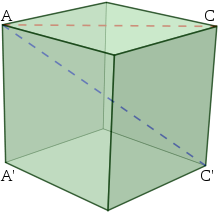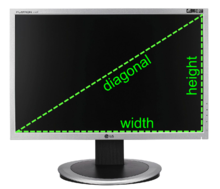# Diagonal

DiagonalThe diagonals of a cube with side length 1. AC' (shown in blue) is a space diagonal with length$\sqrt 3$, while AC (shown in red) is a face diagonal and has length$\sqrt 2$.

A diagonal is a line joining two nonconsecutive vertices of a polygon or polyhedron. Informally, any sloping line is called diagonal. The word "diagonal" derives from the Greek διαγώνιος (diagonios), from dia- ("through", "across") and gonia ("angle", related to gony "knee"); it was used by both Strabo and Euclid to refer to a line connecting two vertices of a rhombus or cuboid, and later adopted into Latin as diagonus ("slanting line").

In mathematics, in addition to its geometric meaning, a diagonal is also used in matrices to refer to a set of entries along a diagonal line.

## Non-mathematical usesA stand of basic scaffolding on a house construction site, with diagonal braces to maintain its structure.

In engineering, a diagonal brace is a beam used to brace a rectangular structure (such as scaffolding) to withstand strong forces pushing into it; although called a diagonal, due to practical considerations diagonal braces are often not connected to the corners of the rectangle.

Diagonal pliers are wire-cutting pliers whose cutting edges intersect the joint rivet at an angle or "on a diagonal".

A diagonal lashing is a type of lashing used to bind spars or poles together applied so that the lashings cross over the poles at an angle.

In association football, the diagonal system of control is the method referees and assistant referees use to position themselves in one of the four quadrants of the pitch.The diagonal is a common measurement of display size.

## Polygons

As applied to a polygon, a diagonal is a line segment joining any two non-consecutive vertices. Therefore, a quadrilateral has two diagonals, joining opposite pairs of vertices. For any convex polygon, all the diagonals are inside the polygon, but for re-entrant polygons, some diagonals are outside of the polygon.

Any n-sided polygon (n ≥ 3), convex or concave, has$\frac{n^2-3n}{2}\,$

diagonals, as each vertex has diagonals to all other vertices except itself and the two adjacent vertices, or n − 3 diagonals.

Sides Diagonals
3 0
4 2
5 5
6 9
7 14
8 20
9 27
10 35
Sides Diagonals
11 44
12 54
13 65
14 77
15 90
16 104
17 119
18 135
Sides Diagonals
19 152
20 170
21 189
22 209
23 230
24 252
25 275
26 299
Sides Diagonals
27 324
28 350
29 377
30 405
31 434
32 464
33 495
34 527
Sides Diagonals
35 560
36 594
37 629
38 665
39 702
40 740
41 779
42 819

## Matrices

In the case of a square matrix, the main or principal diagonal is the diagonal line of entries running from the top-left corner to the bottom-right corner. For a matrix A with row index specified by i and column index specified by j, these would be entries Aij with i = j. For example, the identity matrix can be defined as having entries of 1 on the main diagonal and zeroes elsewhere:$\begin{pmatrix} 1 & 0 & 0 \\ 0 & 1 & 0 \\ 0 & 0 & 1 \end{pmatrix}$

The top-right to bottom-left diagonal is sometimes described as the minor diagonal or antidiagonal. The off-diagonal entries are those not on the main diagonal. A diagonal matrix is one whose off-diagonal entries are all zero.

A superdiagonal entry is one that is directly above and to the right of the main diagonal. Just as diagonal entries are those Aij with j = i, the superdiagonal entries are those with j = i + 1. For example, the non-zero entries of the following matrix all lie in the superdiagonal:$\begin{pmatrix} 0 & 2 & 0 \\ 0 & 0 & 3 \\ 0 & 0 & 0 \end{pmatrix}$

Likewise, a subdiagonal entry is one that is directly below and to the left of the main diagonal, that is, an entry Aij with j = i − 1. General matrix diagonals can be specified by an index k measured relative to the main diagonal: the main diagonal has k = 0; the superdiagonal has k = 1; the subdiagonal has k = − 1; and in general, the k-diagonal consists of the entries Aij with j = i + k.

## Geometry

By analogy, the subset of the Cartesian product X×X of any set X with itself, consisting of all pairs (x,x), is called the diagonal, and is the graph of the identity relation. This plays an important part in geometry; for example, the fixed points of a mapping F from X to itself may be obtained by intersecting the graph of F with the diagonal.

In geometric studies, the idea of intersecting the diagonal with itself is common, not directly, but by perturbing it within an equivalence class. This is related at a deep level with the Euler characteristic and the zeros of vector fields. For example, the circle S1 has Betti numbers 1, 1, 0, 0, 0, and therefore Euler characteristic 0. A geometric way of expressing this is to look at the diagonal on the two-torus S1xS1 and observe that it can move off itself by the small motion (θ, θ) to (θ, θ + ε). In general, the intersection number of the graph of a function with the diagonal may be computed using homology via the Lefschetz fixed point theorem; the self-intersection of the diagonal is the special case of the identity function.

## See also

Wikimedia Foundation. 2010.

### Look at other dictionaries:

• Diagonal — Saltar a navegación, búsqueda Para otros usos de este término, véase Diagonal (desambiguación). Una diagonal es todo segmento que une dos vértices no consecutivos de un polígono o de un poliedro. En sentido coloquial, una diagonal es una recta o… …   Wikipedia Español

• Diagonal — – Radio für Zeitgenossen ist eine mehrfach mit Preisen ausgezeichnete Sendung des österreichischen Radiosenders Ö1. Sie wird samstags zwischen 17.05 Uhr und 19 Uhr ausgestrahlt. Erstmals ging Diagonal am 19. Mai 1984 auf Sendung.… …   Deutsch Wikipedia

• diagonal — diagonal, ale, aux [ djagɔnal, o ] adj. • XIIIe; bas lat. diagonalis; de diagonus, gr. diagônios « ligne tracée d un angle à l autre » ♦ Géom. Qui joint deux sommets (d une figure) qui n appartiennent pas au même côté, à la même face. Ligne… …   Encyclopédie Universelle

• diagonal — DIAGONÁL, Ă, diagonali, e, s.f., adj. I. s.f. 1. Segment de dreaptă care uneşte două unghiuri (sau vârfuri) nealăturate ale unui poligon sau două vârfuri ale unui poliedru aflate pe feţe diferite. ♢ loc. adv. În diagonală = pe direcţia unei… …   Dicționar Român

• Diagonal — Di*ag o*nal, a. [L. diagonalis, fr. Gr. ? from to angle; dia through + ? an angle; perh. akin to E. knee: cf. F. diagonal.] (Geom.) Joining two not adjacent angles of a quadrilateral or multilateral figure; running across from corner to corner;… …   The Collaborative International Dictionary of English

• diagonal — Adj erw. fach. (15. Jh., Form 18. Jh.) Entlehnung. Entlehnt aus ml. diagonalis, eigentlich: durch die Winkel , einer Bildung zu gr. diá durch und gr. gōnía Winkel . Die Diagonale ist die Verbindung zwischen zwei Ecken eines Vielecks in gerader… …   Etymologisches Wörterbuch der deutschen sprache

• diagonal — [dī ag′ə nəl, dīag′nəl] adj. [L diagonalis < Gr diagōnios < dia , through + gōnia, an angle, corner: see KNEE] 1. extending between the vertices of any two nonadjacent angles in a polygonal figure or between any two vertices not in the same …   English World dictionary

• Diagonal — Di*ag o*nal, n. 1. A right line drawn from one angle to another not adjacent, of a figure of four or more sides, and dividing it into two parts. [1913 Webster] 2. (Engin.) A member, in a framed structure, running obliquely across a panel. [1913… …   The Collaborative International Dictionary of English

• diagonal — diagonal, ale (di a go nal, na l ) adj. 1°   Terme de géométrie. Qui va d un angle à un autre, dans une figure rectiligne. Ligne diagonale. 2°   S. f. La diagonale, ligne menée d un angle d une figure quelconque, à un des angles non adjacents.… …   Dictionnaire de la Langue Française d'Émile Littré

• Diagonal — Diagonal, IA U.S. city in Iowa Population (2000): 312 Housing Units (2000): 154 Land area (2000): 0.908254 sq. miles (2.352367 sq. km) Water area (2000): 0.000000 sq. miles (0.000000 sq. km) Total area (2000): 0.908254 sq. miles (2.352367 sq. km) …   StarDict's U.S. Gazetteer Places

• Diagonal, IA — U.S. city in Iowa Population (2000): 312 Housing Units (2000): 154 Land area (2000): 0.908254 sq. miles (2.352367 sq. km) Water area (2000): 0.000000 sq. miles (0.000000 sq. km) Total area (2000): 0.908254 sq. miles (2.352367 sq. km) FIPS code:… …   StarDict's U.S. Gazetteer Places

### Share the article and excerpts

##### Direct link
Do a right-click on the link above
and select “Copy Link”